34 Associations
Counts gives an association that reports how many times each different element occurs:
 In:=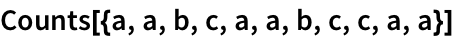Out=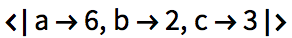Find the value associated with c in the association:
 In:=Out=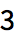Operations that work on lists typically also work on associationsbut apply themselves only to the values, not the keys.
This adds 500 to each value in the association:
 In:=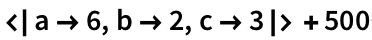Out=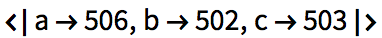/@ applies a function to each value in the association:
 In:=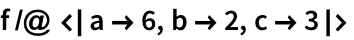Out=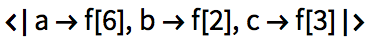Total gives the total of the values:
 In:=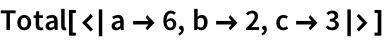Out=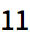Sort operates on the values:
 In:=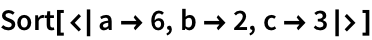Out=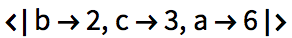KeySort operates on the keys:
 In:=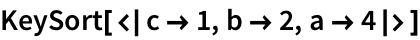Out=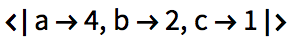The functions Keys and Values extract the keys and values of an association.
Get the list of keys in the association:
 In:=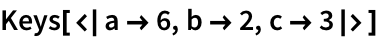Out=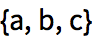Get the list of values in the association:
 In:=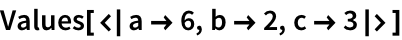Out=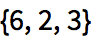Normal turns an association into a normal list of rules. Association makes an association from a list of rules.
 In:=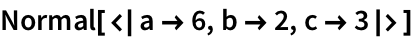Out=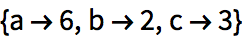In:=Out=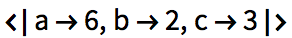LetterCounts counts how many times letters occur in a string.
 In:=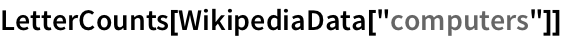Out=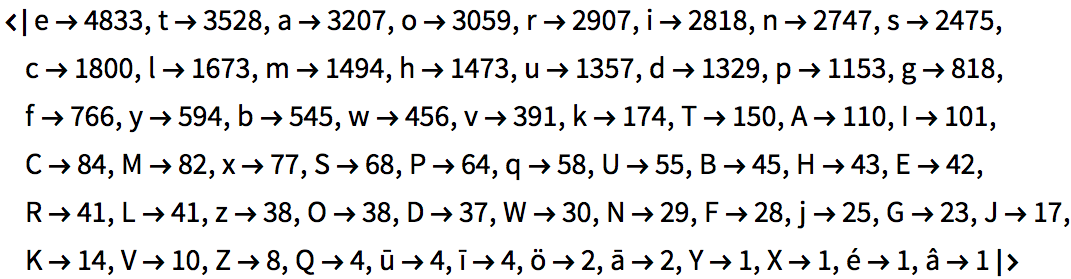KeyTake picks out elements of an association that appear in a list of keys you specify. Here were taking the elements whose keys are letters in the (lowercase) alphabet.
Take only those elements in the association whose keys appear as letters in the alphabet:
 In:=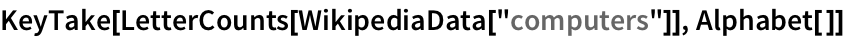Out=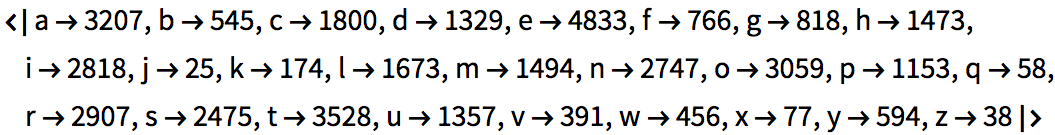BarChart plots the values in an association. With the option , it uses the keys as labels.
Make a bar chart of how many times each letter appears; e is the most common:
 In:=Out=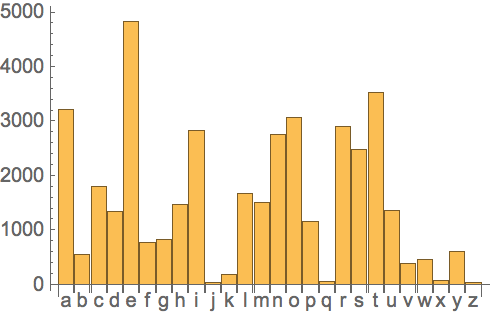Heres a direct way to apply a pure function to an association:
Apply a pure function to an association:
 In:=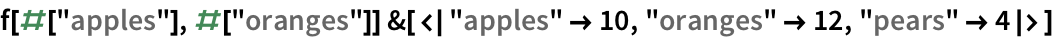Out=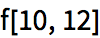Its very common to have keys that are strings, and the Wolfram Language has a special way to handle these when it comes to pure functions: you can just use #key to refer to an element whose key is "key".
Use the simpler notation for association elements whose keys are strings:
 In:=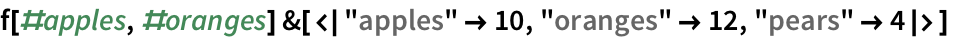Out=As a more realistic example, apply a pure function that extracts the value for e from the letter counts, and divides by the total. The N gives the result as a decimal.
 In:=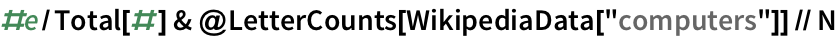Out=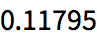key1value1,key2value2, ... an association Association[rules] turn a list of rules into an association assoc[key] extract an element of an association Keys[assoc] list of keys in an association Values[assoc] list of values in an association Normal[assoc] turn an association into a list of rules KeySort[assoc] sort an association by its keys KeyTake[assoc,keys] take elements with particular keys #key function slot for an element with key “key” Counts[list] an association with counts of distinct elements LetterCounts[string] an association with counts of distinct letters
34.1Make a list, in order, of the number of times each of the digits 0 through 9 occurs in 3^100. »
Expected output:
 Out[]=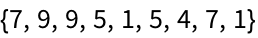Answer & check your solution
34.2Make a labeled bar chart of the number of times each of the digits 0 through 9 occurs in 2^1000. »
Expected output:
 Out[]=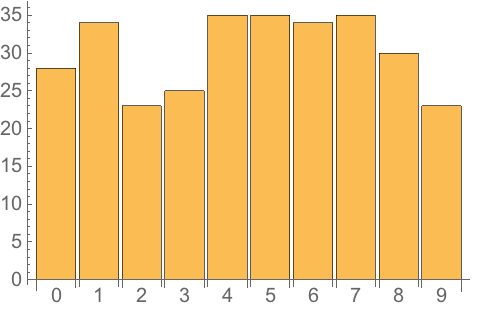Answer & check your solution
34.3Make a labeled bar chart of the number of times each possible first letter occurs in words from WordList[ ]»
Expected output:
 Out[]=Answer & check your solution
34.4Make an association giving the 5 most common first letters of words in WordList[ ] and their counts. »
Sample expected output:
 Out[]=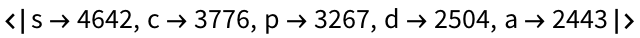Answer & check your solution
34.5Find the numerical ratio of the number of occurrences of q and u in the Wikipedia entry for computers. »
Sample expected output:
 Out[]=Answer & check your solution
34.6Find the 10 most common words in ExampleData[{"Text", "AliceInWonderland"}]»
Expected output:
 Out[]=Answer & check your solution
Because they associate values with keys. Other names used for the same concept are associative arrays, dictionaries, hashmaps, structs, key-value maps and symbolically indexed lists.
How does one type in an association?
Start with <| (< followed by |), then use -> for each . Alternatively use Association[a->1, b->2].
Can an association have several elements with the same key?
No. Keys in an association are always unique.
Normally you get Missing[...]. But if you use Lookup to look up the key, you can specify what to return if the key is absent.
Use KeyMap, or use functions like KeySelect and KeyDrop. AssociationMap creates an association by mapping a function over keys.
Use Merge. You have to give a function to say what to do if the same key occurs in multiple associations.
Can one use [[...]] to extract part of an association, like one extracts part of a list?
Yes, if you explicitly say assoc[[Key[key]]]. For example, assoc[] will extract the second element of assoc, whatever key it has. assoc[[key]] is a special case that works the same as assoc[key].
What happens in pure functions if the keys in an association arent strings?
You cant use #key anymore; you have to explicitly use #[key].